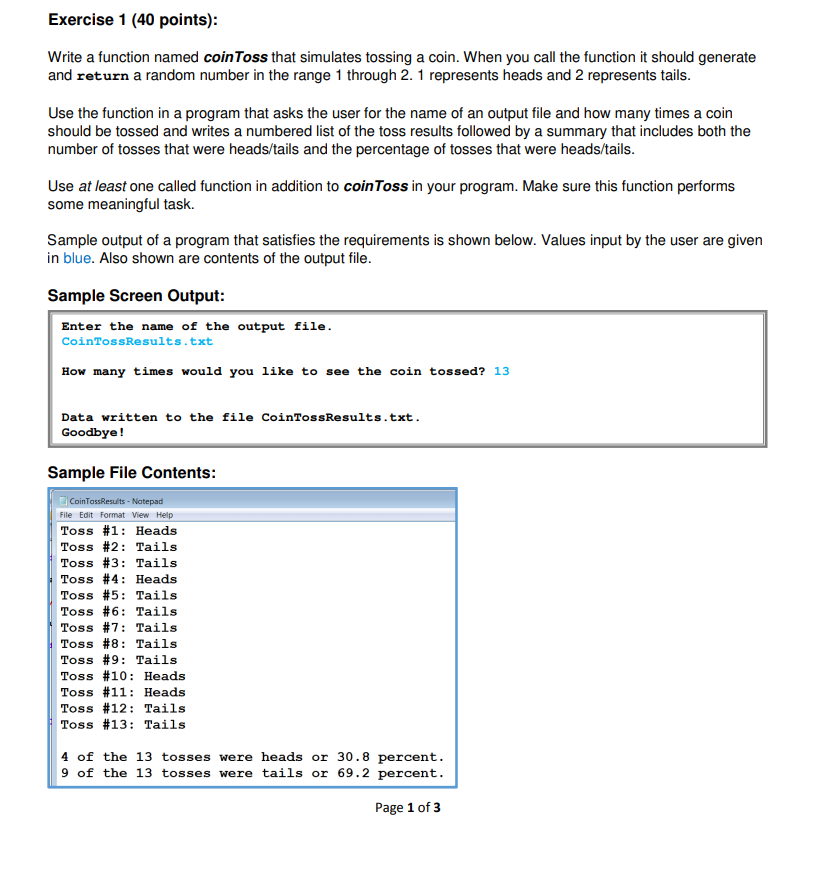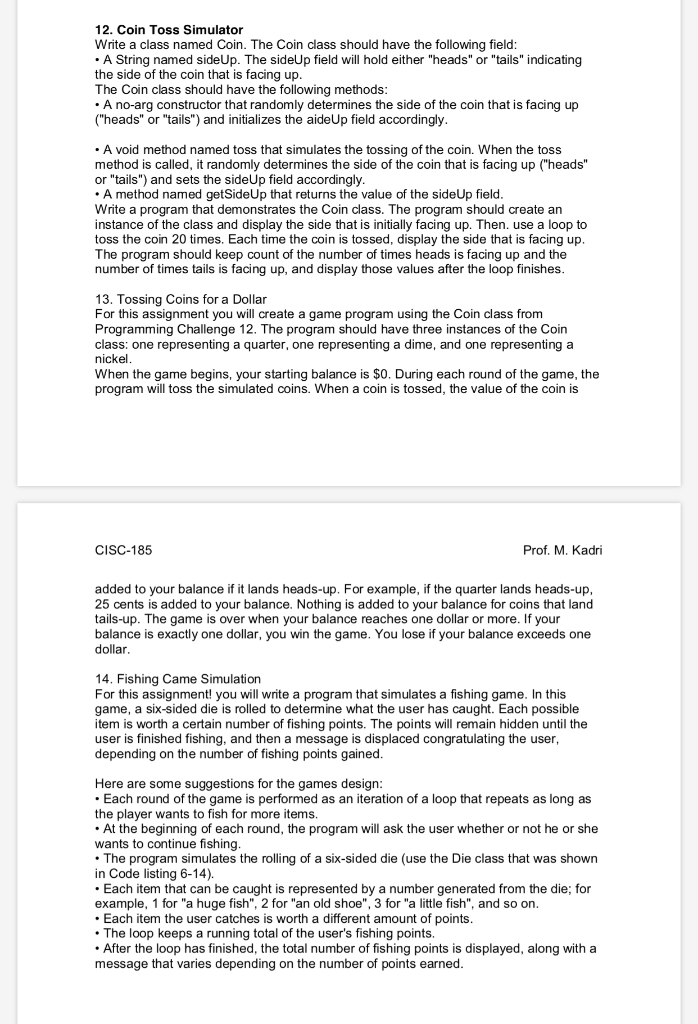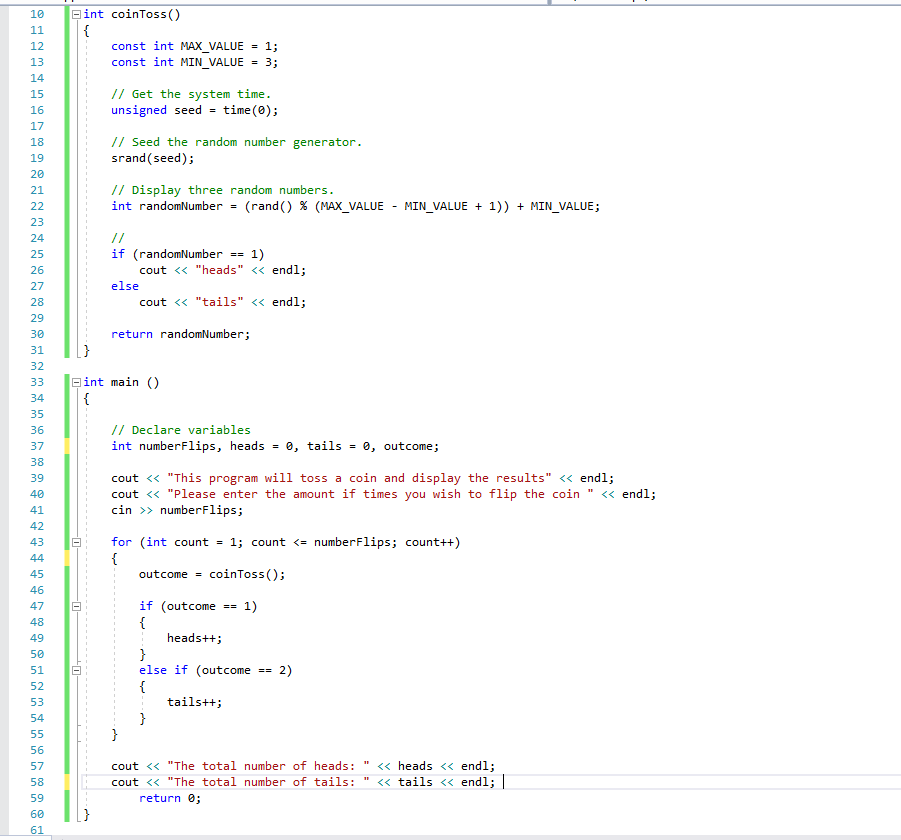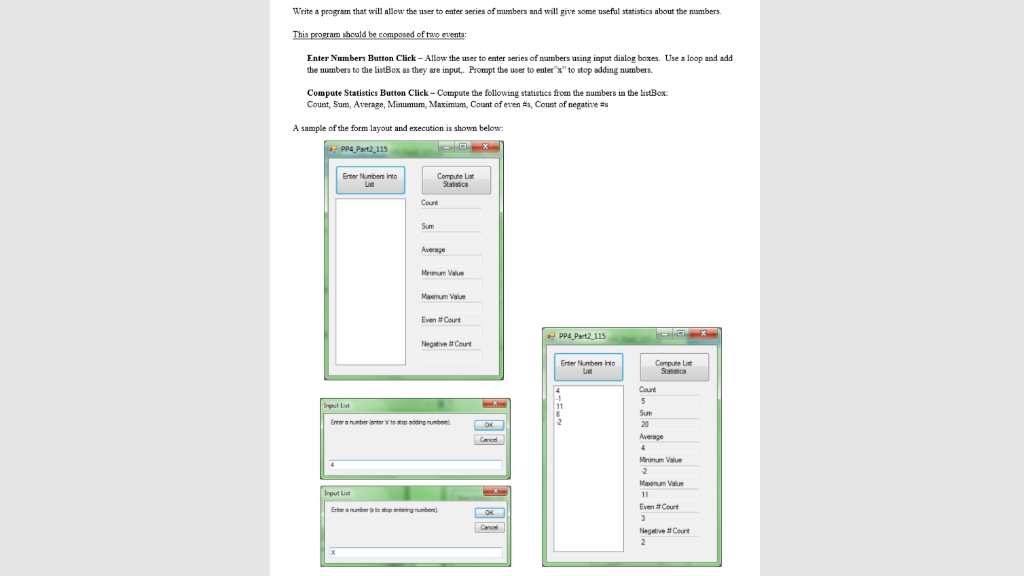# Write an algorithm that will compute statistics for 8 coin tosses. the user will enter either...

Write an algorithm that will compute statistics for 8 coin tosses. the user will enter either an h for heads or t for tails for the eight tosses. The program will then display the total number and percentages of heads and tails. Use the increment operator to count each h and t that is entered.

Start.

1. initialize counth=0 and count=0.

2. for toss=1 to toss=8 do

input value;

if value=='h'

increase counth as counth++;

else

if value == 't'

increase count as count++;

3. Calculate total head percentage as counth*100/8

4. Calculate total tail percentage as count*100/8

5. Display the result

End.

#### Earn Coin

Coins can be redeemed for fabulous gifts.

Similar Homework Help Questions
• ### Coin Toss Simulator. C++ Write a class named Coin. The Coin class should have the following...

Coin Toss Simulator. C++ Write a class named Coin. The Coin class should have the following member variables: A string named sideUp. The sideUp member variable will holder either "heads" or "tails" indicating the side of the coin that is facing up. The Coin class should have the following member functions: A default constructor that randomly determines the side of the coin that is facing up ("heads" or "tails") and initializes the sideUp member variable accordingly. A void member function...

• ### Using c++ Write a function named coinToss that simulates tossing a coin. When you call the...Using c++ Write a function named coinToss that simulates tossing a coin. When you call the function it should generate and return a random number in the range 1 through 2. 1 represents heads and 2 represents tails. Exercise 1 (40 points) Write a function named coinToss that simulates tossing a coin. When you call the function it should generate and return a random number in the range 1 through 2.1 represents heads and 2 represents tails Use the function...

• ### 12. Coin Toss Simulator Write a class named Coin. The Coin class should have the following field ...12. Coin Toss Simulator Write a class named Coin. The Coin class should have the following field .A String named sideUp. The sideUp field will hold either "heads" or "tails" indicating the side of the coin that is facing up The Coin class should have the following methods .A no-arg constructor that randomly determines the side of the coin that is facing up (heads" or "tails") and initializes the aideUp field accordingly .A void method named toss that simulates the...

• ### Heads and Tails C programming variable length arrays.

Write a program that prompts the user to toss a coin a set number of times and then determines how many times heads and tails were flipped.The program should prompt the user to enter a random seed. Use “Enter a random seed: ” as the prompt. After reading the random seed, use it seed the random numbergenerator. Then prompt the user to enter the number of coin tosses. Use “Enter the number of coin tosses: ” as the prompt. Use...

• ### In C++ please Create a coin-flipping game. Ask the user how many times to flip the...

In C++ please Create a coin-flipping game. Ask the user how many times to flip the coin, and use the random function to determine heads or tails each time a coin is flipped. Assume the user starts with \$50. Every time the coin is flipped calculate the total (heads +\$10, tails -\$10). Create another function to test if the user has gone broke yet (THIS FUNCTION MUST RETURN A BOOLEAN TRUE/FALSE VALUE). End the program when the user is broke...

• ### Problem: Write a function named coinToss that simulates the tossing of a coin. When you call the function, it should ge...Problem: Write a function named coinToss that simulates the tossing of a coin. When you call the function, it should generate a random number in the range of 1 through 2. If the random number is 1, the function should display “heads.” If the random number is 2, the function should display “tails.” Demonstrate the function in a program that asks the user how many times the coin should be tossed and then simulates the tossing of the coin that...

• ### Write a C program that prompts the user for the number for times the user wants...

Write a C program that prompts the user for the number for times the user wants to flip a coin. Your program will flip a virtual coin that number of times and return the following: 1) The number of times heads occurred. 2) The number of times tails occured. Then take the coin flip program and modify the random number generator to roll a single 6-sided dice.   DO NOT DISPLAY THE RESULTS OF EACH ROLL. 1) Ask the user how...

• ### c++ Program 2: Coin Toss Simulator For this program, please implement Problems 12 and 13 in...

c++ Program 2: Coin Toss Simulator For this program, please implement Problems 12 and 13 in a single program (Gaddis, p812, 9E). Scans of these problems are included below. Your program should have two sections that correspond to Problems 12 and 13: 1) As described in Problem 12, implement a Coin class with the specified characteristics. Run a simulation with 20 coin tosses as described and report the information requested in the problem. 2) For the second part of your...

• ### "Flip a Coin!" You will write a program that allows the user to simulate a coin...

"Flip a Coin!" You will write a program that allows the user to simulate a coin flip. Note the following line of code: (Math.random()*2) +1; This line generates a random number (a float) between 1 and 2. If you wanted to simulate somebody rolling a die, you could store the value of this variable as: var coinFlip = (Math.random()*2) +1; (Be sure to include all the parentheses in this line of code.) Reminder - Of course, you’ll need to convert...

• ### write a programming code for the following problem using Visual Basic Studio VB. Write a program that will allow the user to enter series of mumbers and will give some useful statistics about the mam...write a programming code for the following problem using Visual Basic Studio VB. Write a program that will allow the user to enter series of mumbers and will give some useful statistics about the mambers Enter Numbers Button Click-Allow the user to enter series of nambers using imput dialog boxes. Use a loop and add the numbers to the listBox as they are input.. Prompt the user to enter"x" to stop addang numbers. Compute Statistics Button Click-Compute the following statistics...